NCERT Solutions for Class 6 Maths Chapter 14 Practical Geometry Ex 14.5 are part of NCERT Solutions for Class 6 Maths. Here we have given NCERT Solutions for Class 6 Maths Chapter 14 Practical Geometry Ex 14.5.

 Board CBSE Textbook NCERT Class Class 6 Subject Maths Chapter Chapter 14 Chapter Name Practical Geometry Exercise Ex 14.5 Number of Questions Solved 9 Category NCERT Solutions

## NCERT Solutions for Class 6 Maths Chapter 14 Practical Geometry Ex 14.5

Question 1.
Draw $$\overline { AB }$$ of length 7.3 cm and find its axis of symmetry.
Solution :
Step 1. Draw a line segment $$\overline { AB }$$ of length 7.3 cm.
Step 2. With A ascentere, using compasses, drawthe circle. The radius of this circle should be more than half of the length of $$\overline { AB }$$.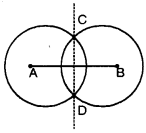Step 3. With the same radius and with B as a centre, draw another circle using compasses. Let it cut the previous circle at C and D.
Step 4. Join CD. Then, $$\overline { CD }$$ is the axis of symmetry of $$\overline { AB }$$.

Question 2.
Draw a line segment of length 9.5 cm and construct its perpendicular bisector.
Solution :
Step 1. Draw a line segment $$\overline { AB }$$ of length 9.5 cm.Step 2. With A as a centre, using compasses, draw a circle. The radius of this circle should be more than half of the length of $$\overline { AB }$$.
Step 3. With the same radius and with B as a centre, draw another circle using compasses. Let it cut the previous circle at C and D.
Step 4. Join CD. Then $$\overline { CD }$$ is the perpendicular bisector of the line segment $$\overline { AB }$$.

Question 3.
Draw the perpendicular bisector of $$\overline { XY }$$ whose length is 10.3 cm.
(a) Take any point P on the bisector drawn. Examine whether PX = PY.
(b) If M is the midpoint of $$\overline { XY }$$, what can you say about the lengths MX and XY ?
Solution :
Step 1. Draw a line segment $$\overline { XY }$$ of length 10.3 cm.
Step 2. With X as a centre, using compasses, draw a circle. The radius of this circle should be more than half of the length of $$\overline { XY }$$.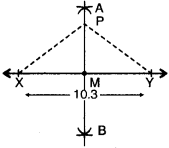Step 3. With the same radius and with Y as a centre, draw another circle using compasses. Let it cuts the previous circle at A and B.
Step 4. Join AB. Then $$\overline { AB }$$ is the perpendicular bisector of the line segment $$\overline { XY }$$.
(a) On examination, we find that PX = PY.
(b) We can say that the lengths of MX is half of the length of XY.

Question 4.
Draw a line segment of length 12.8 cm. Using compasses, divide it into four equal parts. Verify by actual measurement.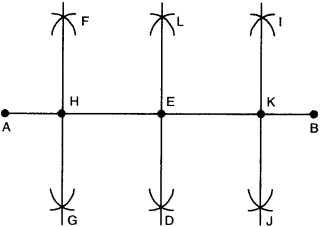Solution :
Step 1. Draw a line segment $$\overline { AB }$$ of length 12.8 cm.
Step 2. With A as centre, using compasses, draw two arcs on either side of AB. The radius of this arc should be more than fialf of the length of $$\overline { AB }$$.
Step 3. With the same radius and with B as centre, draw another arcs using compasses. Let it cut the previous arcs at C and D.
Step 4. Join $$\overline { CD }$$. It cuts $$\overline { AB }$$ at E. Then $$\overline { CD }$$ is the perpendicular bisector of the line segment $$\overline { AB }$$.
Step 5. With A as centre, using compasses, draw a circle. The radius of this circle should be more than half of the length of AE.
Step 6. With the same radius and with E as centre, draw another circle using compasses; Let it cut the previous circle at F and G.
Step 7. Join $$\overline { FG }$$. It cuts $$\overline { AE }$$ at H. Then $$\overline { FG }$$ is the perpendicular bisector of the line segment $$\overline { AE }$$.
Step 8. With E as centre, using compasses, draw a circle. The radius of this circle should be more than half of the length of EB.
Step 9. With the same radius and with B as centre, draw another circle using compasses. Let it cut the previous circle at I and J.
Step 10. Join $$\overline { IJ }$$. It cuts $$\overline { EB }$$ at K. Then $$\overline { IJ }$$ is the perpendicular bisector of the line segment $$\overline { EB }$$. Now, the points H, E and K divide AB into four equal parts, i.e., $$\overline { AH }$$ = $$\overline { HE }$$ = $$\overline { EK }$$ = $$\overline { KB }$$ By measurement, $$\overline { AH }$$ = $$\overline { HE }$$ = $$\overline { EK }$$ = $$\overline { KB }$$ = 3.2 cm.

Question 5.
With $$\overline { PQ }$$ of length 6.1 cm as diameter draw a circle.
Solution :
Step 1. Draw a line segment $$\overline { PQ }$$ of length 6.1 cm.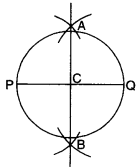Step 2. With P as centre, using compasses, draw a circle. The radius of this circle should be more than half of the length of $$\overline { PQ }$$.
Step 3. With the same radius and with Q as centre, draw another circle using compasses. Let it cut the previous circle at A and B.
Step 4. Join $$\overline { AB }$$. It cuts $$\overline { PQ }$$ at C. Then $$\overline { AB }$$ is
the perpendicular bisector of the line segment PQ .
Step 5. Place the pointer of the compasses at C and open the pencil upto P.
Step 6. Turn the compasses slowly to draw the circle.

Question 6.
Draw a circle with centre C and radius, 3.4 cm. Draw any chord $$\overline { AB }$$. Construct the perpendicular bisector of $$\overline { AB }$$ and examine if it passes through C.
Solution :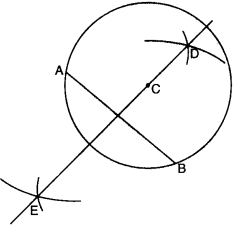Step 1. Draw a point with a sharp pencil and mark it as C.
Step 2. Open the compasses for the required radius 3.4 cm, by putting the pointer on 0 and opening the pencil upto 3.4 cm.
Step 3. Place the pointer of the compasses at C. Step 4. Turn the compasses slowly to draw the
circle.
Step 5. Draw any chord $$\overline { AB }$$ of this circle.
Step 6. With A as centre, using compasses, draw a circle. The radius of this circle should be more than half of the length of $$\overline { AB }$$.
Step 7. With the same radius and with B as centre, draw another circle using compasses. Let it cut the previous circle at D and E.
Step 8. Join $$\overline { DE }$$ . Then $$\overline { DE }$$ is the perpendicular bisector of the line segment $$\overline { AB }$$. On examination, we find that it passes through C.

Question 7.
Repeat Question 6, if $$\overline { AB }$$ happens to be a diameter.
Solution :
Step 1. Draw a point with a sharp pencil and mark it as C.
Step 2. Open the compasses for the required radius 3.4 cm, by putting the pdinter of compasses on 0 of the scale and opening the pencil upto 3.4 cm.
Step 3. Place the pointer of the compasses at C.
Step 4. Turn the compasses slowly to draw the circle.
Step 5. Draw any diameter $$\overline { AB }$$.Step 6. With A as centre, using compasses, draw arcs on either side. The radius of this arc should be more than half of the length of $$\overline { AB }$$.
Step 7. With the same radius and with B as centre, draw another arcs using compasses. Let it cut the previous arcs at D and E.
Step 8. Join $$\overline { DE }$$ . Then $$\overline { DE }$$ is the perpendicular bisector of the line segment $$\overline { AB }$$. On examination, we find that it passes through C.

Question 8.
Draw a circle of radius 4 cm. Draw any two of its chords. Construct the perpendicular bisectors of these chords. Where do they meet?
Solution :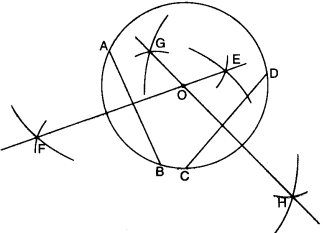Step 1. Draw a point with a sharp pencil and mark it as O.
Step 2. Open the compasses for the required radius of 4 cm. by putting the pointer on 0 and opening the pencil upto 4 cm.
Step 3. Place the pointer of the compasses at O.
Step 4. Turn the compasses slowly to draw the circle.
Step 5. Draw any two chords $$\overline { AB }$$ and $$\overline { CD }$$ of this circle.
Step 6. With A as centre, using compasses, draw two arcs on either side of AB. The radius of this arc should be more than half of the length of $$\overline { AB }$$.
Step 7. With the same radius and with B as centre, draw another two arcs using compasses. Let it cut the previous circle at E and F.
Step 8. Join $$\overline { EF }$$. Then $$\overline { EF }$$ is the perpendicular bisector of the chord $$\overline { AB }$$.
Step 9. With C a< centre, using compasses, draw two arcs on either side of CD. The radius of this arc should be more than half of the length of $$\overline { CD }$$.
Step 10. With the same radius and with D as centre, draw another two arcs using compasses. Let it cut the previous circle at G and H.
Step 11. Join $$\overline { GH }$$. Then $$\overline { GH }$$ is the perpendi¬cular bisector of the chord $$\overline { CD }$$. We find that the perpendicular bisectors $$\overline { EF }$$ and $$\overline { GH }$$ meet at O, the centre of the circle.

Question 9.
Draw any angle with vertex O. Take a point A on one of its arms and B on another such that OA- OB. Draw the perpendicular bisectors of $$\overline { OA }$$ and $$\overline { OB }$$. Let them meet at P. Is PA = PB?
Solution :
Step 1. Draw any angle POQ with vertex O.
Step 2. Take a point A on the arm OQ and another point B on the arm OP such that $$\overline { OA }$$ = $$\overline { OB }$$.
Step 3. With O as centre, using compasses, draw a circle. The radius of this circle should be more than half of the length of $$\overline { OA }$$.Step 4. With the same radius and with A as centre, draw another circle using compasses. Let it cut the previous circle at C and D.
Step 5. Join $$\overline { CD }$$. Then $$\overline { CD }$$ is the perpendicular bisector of the line segment $$\overline { OA }$$.
Step 6. With O as centre, using compasses, draw a circle. The radius of this circle should be more than half of the length of $$\overline { OB }$$.
Step 7. With the same radius and with B as centre, draw another circle using compasses. Let it cut the previous circle at E and F.
Step 8. Join $$\overline { EF }$$. Then $$\overline { EF }$$ is the perpendicular bisector of the line segment OB. The two perpendicu¬lar bisectors meet at P.
Step 9. Join $$\overline { PA }$$ and $$\overline { PB }$$. We find that $$\overline { PA }$$ = $$\overline { PB }$$.

We hope the NCERT Solutions for Class 6 Maths Chapter 14 Practical Geometry Ex 14.5 help you. If you have any query regarding NCERT Solutions for Class 6 Maths Chapter 14 Practical Geometry Ex 14.5, drop a comment below and we will get back to you at the earliest.